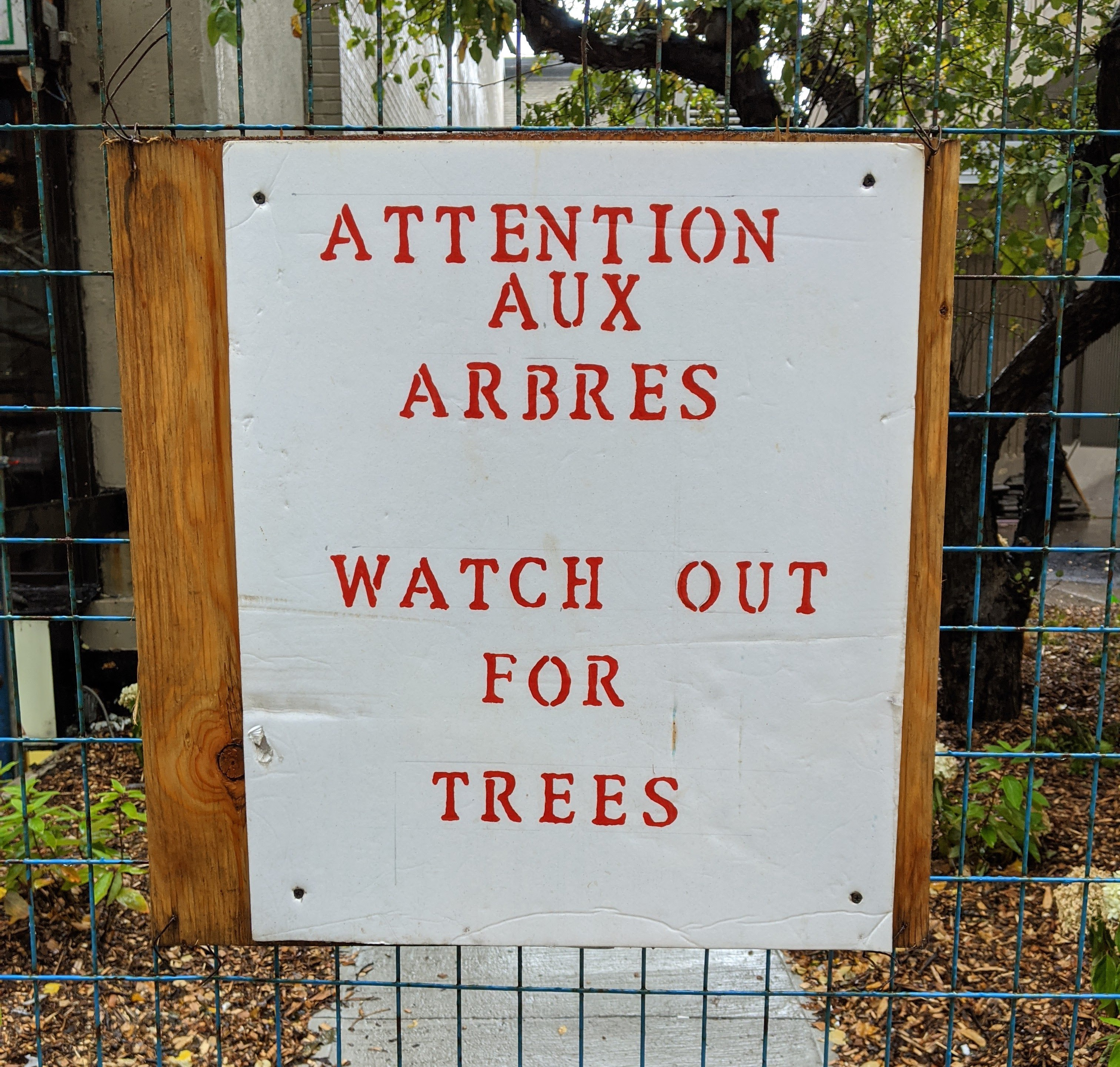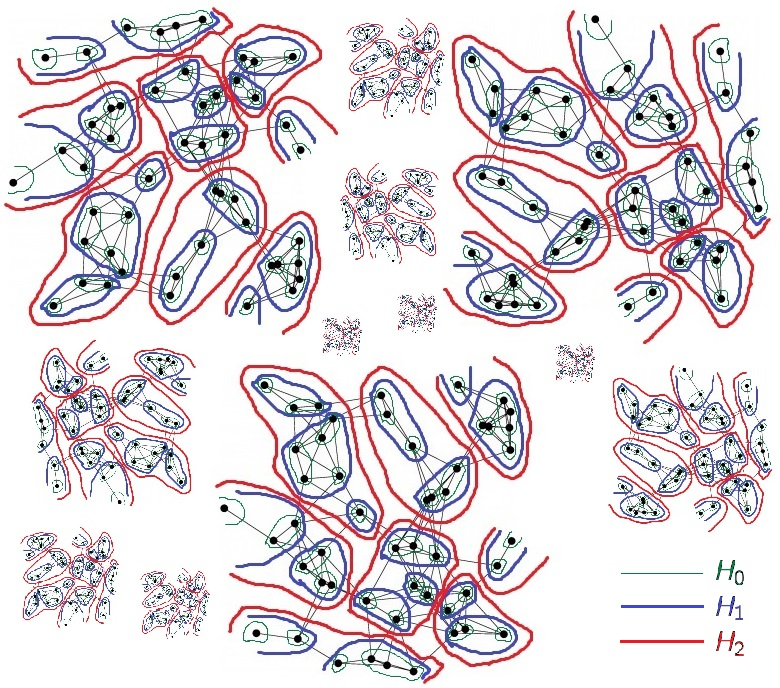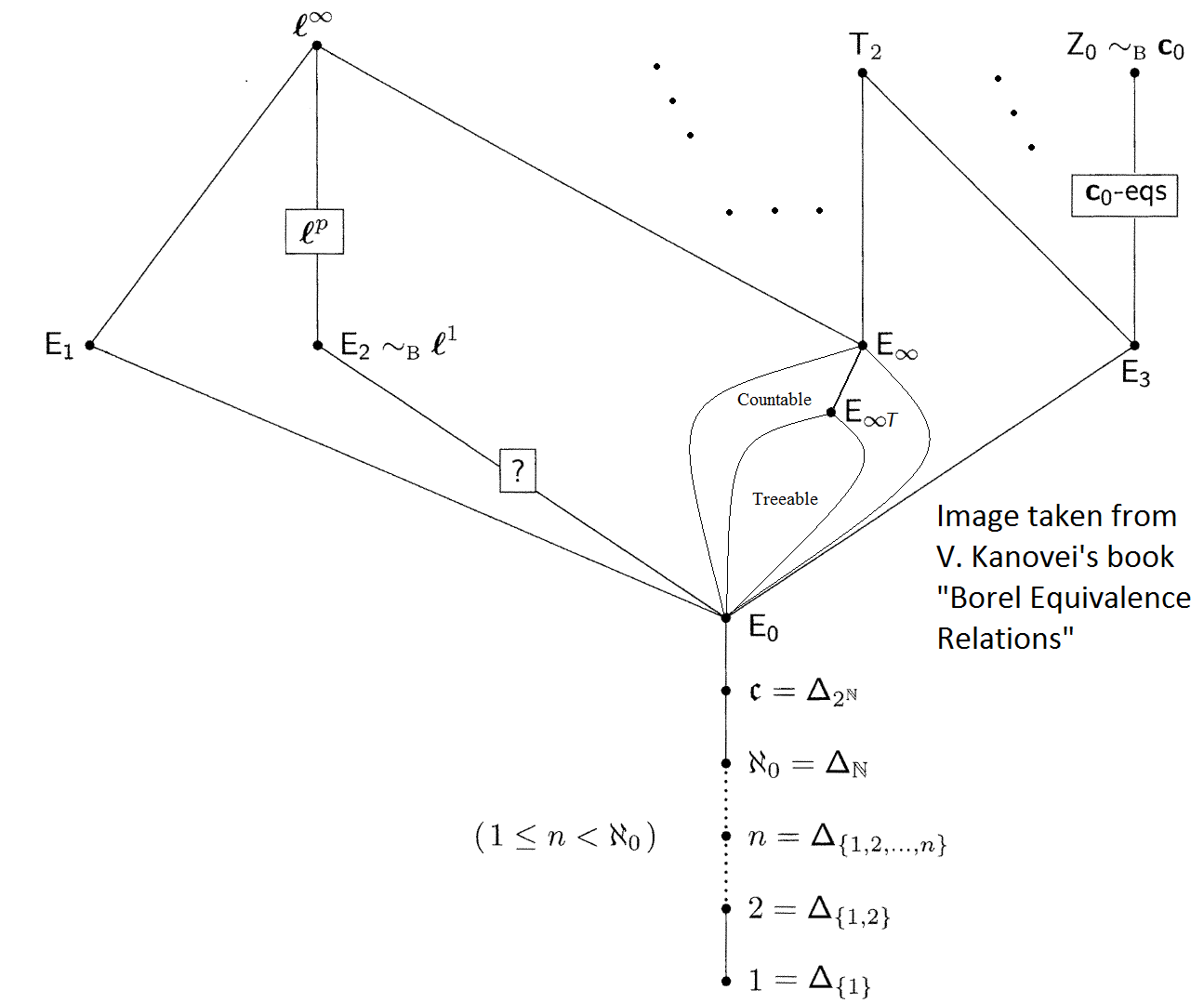# Անուշ ԾերունյանMy research is rooted in logic and descriptive set theory with outcomes in ergodic theory, measured group theory, combinatorics, and geometric group theory.

### Papers

• A backward pointwise ergodic theorem and its forward implications [arXiv] (2020+)
▻with Jenna Zomback
• Characterization of saturated graphs related to pairs of disjoint matchings [arXiv] (2020+)
▻with Zhengda Mo, Samuel Qunell, and Jenna Zomback
• Pairs of disjoint matchings and related classes of graphs [arXiv] (2020+)
▻with A. Guo, K. Kaempen, Z. Mo, S. Qunell, J Rogge, C. Song, J. Zomback
• The ratio ergodic theorem [pdf] (2020)
▻with Benjamin Miller
• A descriptive construction of trees and Stallings' theorem [arXiv]
Trends in set theory, Contemporary Mathematics, vol. 752 (2020), Amer. Math. Soc., Providence, RI, pp. 191–207
• Independent sets in algebraic hypergraphs [pdf, arXiv]
▻with Anton Bernshteyn and Michelle Delcourt
to appear in Journal of the European Math. Soc. (2019+)
• A short nonalgorithmic proof of the containers theorem for hypergraphs [pdf, arXiv, DOI]
▻with Anton Bernshteyn, Michelle Delcourt, and Henry Towsner
Proceedings of the American Math. Soc., vol. 147 (2019), pp. 1739–1749
• Pointwise ergodic theorem for locally countable quasi-pmp graphs [pdf, arXiv] (2018+)
• A descriptive set theorist's proof of the pointwise ergodic theorem [pdf, arXiv] (2017)
• Edge sliding and ergodic hyperfinite decomposition [pdf, arXiv] (2017+)
▻with Benjamin Miller
• Mixing and double recurrence in probability groups [pdf, arXiv] (2014, 2017+)
•• A Ramsey theorem on semigroups and a general van der Corput lemma [pdf, arXiv, DOI]
Journal of Symbolic Logic, vol. 81 (2016), no. 2, pp. 718–741
• Finite generators for countable group actions in the Borel and Baire category settings [pdf, arXiv, DOI]
Advances in Mathematics, vol. 269 (2015), pp. 585–646
• Characterization of a class of graphs related to pairs of disjoint matchings [pdf, arXiv, DOI]
Discrete Mathematics, vol. 309 (2009), no. 4, pp. 693–713
• On edge-disjoint pairs of matchings [pdf, arXiv, DOI]
▻with Vahan Mkrtchyan and Vahé Musoyan
Discrete Mathematics, vol. 308 (2008), no. 23, pp. 5823–5828

### Work in preparation

• The structure of hyperfinite equivalence relations in a quasi-pmp acyclic graph (2020+)
▻with Robin Tucker-Drob
• Topological dimension and Baire category in Euclidean spaces
• Finite index subequivalence relations and treeability

### Ph.D. Thesis

Finite generators for countable group actions; Finite index pairs of equivalence relations; Complexity measures for recursive programs [pdf, ProQuest]
UCLA (May, 2013)### Other writing

The following are some things I've written that are either partially published or not (currently) intended for publication.
• Realizations of graphs in a Hilbert space [pdf]
(with Terence Tao) Banff International Research Station, Canada (July, 2015)
• The Effros space of a σ-Polish space is standard Borel [pdf]
UIUC (January, 2014)
• Countable compact Hausdorff spaces are Polish [pdf]
UCLA (March, 2013)
• Segal's effective witness to measure-hyperfiniteness [pdf]
Caltech (Fall, 2012)
• Hjorth's proof of the embeddability of hyperfinite equivalence relations into E0 [pdf]
UCLA (Fall, 2010)

### Contributions in others' works

• Y. Moschovakis, Abstract Recursion and Intrinsic Complexity, to appear in the ASL Lecture Notes in Logic (2018) [pdf]
Section 3B consists of the results of Part 3 of my Ph.D. thesis on complexity measures for recursive programs.
UCLA (Fall, 2009)
• A. Kechris, The spaces of measure preserving equivalence relations and graphs, preprint (2017) [pdf]
Sections 3, 6, 19 contain some of my results regarding the weak and strong topologies, the discontinuity of the map from actions to equivalence relations, and subtreeings of treeings of an equivalence relation.
Caltech (Spring, 2013)
• M. Lupini, Polish groupoids and functorial complexity, Trans. of the Amer. Math. Soc., 369 (2017), no. 9, 6683–6723 [arXiv]
Appendix consists of my proof of the Effros space of a σ-Polish space being standard Borel. [pdf]
UIUC (January, 2014)